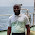## Wednesday, 10 January 2018

### Multicollinearity

Multicollinearity, pronounced as mul-ti-co-lli-nea-ri-ty is the second longest word in the econometrics dictionary after heteroscedasticity. It contains 17 talking words! It occurs when there exists perfect or exact linear dependence or relationships among explanatory variables in a given model. Collinearity is when such exact dependence is between two variables. In that wise, we say that the variables are collinear. For instance, when an explanatory variable is 80 to 100% explained by another explanatory variable, separating the influence of each of them on the dependent variable (regressand) becomes difficult and interpreting the estimated coefficients from that model will also be problematic. This is because variation in one regressor can be completely explained by another regressor in the same model.

With perfect or less than perfect multicollinearity or collinearity:

Ø  Regression coefficients are indeterminate (because the collinear variables cannot be distinguished from one another)

Ø  Standard errors are infinite (they are very large)

Ø  Estimates are biased

Ø  Coefficients cannot be estimated with precision or accuracy

Note: multicollinearity does not violate any regression assumptions; the OLS estimators are still BLUE (Best Linear Unbiased Estimators); it does not destroy the property of minimum variance.

Multicollinearity can be detected using “r” the coefficient of correlation. So, if r = 1, then multicollinearity or collinearity exists. So whenever you run your correlation matrix, look out for those relationship where r > 0.8, that tells us that the respective variables are collinear. Multicollinearity is ruled out when regressors in a model have non-linear relationships.

A major problem associated with multicollinearity is that, if r is high, then the standard error will be high and the computed t-statistic will be low making it more likely not to reject the null hypothesis when is false. Thereby committing a Type II error….that is, incorrectly retaining a very false null hypothesis.

How do you know if your model suffers from multicollinearity?

Ø  High R2

Ø  Few significant t-ratios

Ø  Wider confidence intervals

Ø  Contradictory signs of beta coefficients to expected a priori

Ø  Estimates are sensitive to even small changes in model specification

Ø  High pair-wise correlation statistic among the regressors

Ø  From the tolerance level and variance inflation factor (VIF). A tolerance level lower than 0.10 and a VIF of 10 are indicative of multicollinearity in a model.  A higher VIF provides evidence of multicollinearity.

Correcting/controlling for multicollinearity:

Ø  Collect more data

Ø  Change the scope of analysis

Ø  Do not include collinear variables in the same regression

Ø  Drop the highly collinear variable

Ø  Transform the collinear variable through differencing (however, the differenced error term is serially-correlated and violates OLS assumptions).

What I do often, is to drop the collinear variable and if that variable is very important to my model, I’ll transform my modelling structure into a step-wise fashion such that collinear variables are not included together in the same regression.

[Watch video on multicollinearity]

Post your comments and questions….

Back to Home

#### 4 comments:

1.Great article.
However, the first (and hilarious) rule of correcting for the presence of multicollinearity is to "DO NOTHING". The importance of this, I guess, is for the researcher to take his/her time to re-observe the model, data and try to fish out what might be the problem, at first glance!
Having observed and found no immediate faults then your step 1 suffices, and so on....

1.Yes, I couldn't agree more. Blanchard (1967) coined the "do nothing" statement when students are frantic to conclude that their OLS analysis is wrong whenever multicollinearity is observed in the model. But since multicollinearity is essentially a data efficiency problem it is important to treat it using scientific means - the tolerance level (TOL) or VIF approach. if TOL is lower then 0.10 or VIF greater than 10, then multicollinearity is present. Be that at it may, no OLS property is violated and the estimators are still BLUE.

2.Great Job ma! Does this best explains situations where the researcher has gathered data either from a wrong source. Or there's a deliberate uniformity among respondent to falsify or manipulate the management decision.

1.Well there is no direct answer to this because no one can actually say which data source is genuine or whether your respondents will be truthful when filling your questionnaires. However, it now becomes the researcher's responsibility to sift through the data, perform the pre-estimation procedures which includes conducting multicollinearity tests to observed the relationships between or among the explanatory variables. This becomes necessary for informed policy making decisions.